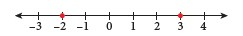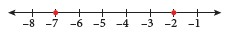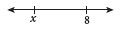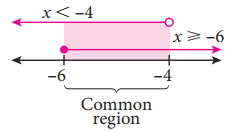## Linear Inequalities

7.1  Inequalities

 Definition The relationship between two quantities that do not have the same value.

 Symbol Meaning $$\gt$$ Greater than $$\lt$$ Less than

 ExampleFrom the number line above, $$–2$$ lies to the left of $$3$$. So, $$–2$$ is less than $$3$$. Thus, the inequality is $$–2 < 3$$.

 ExampleFrom the number line above, $$–2$$ lies to the right of $$-7$$. So, $$–2$$ is greater than $$-7$$. Thus, the inequality is $$–2 > -7$$.

Describe inequality and form algebraic inequality:

 ExampleFrom the number line above, $$x$$ is less than $$8$$. Thus, $$x\lt 8$$.

Identify relationship:

 Symbol Meaning $$\geq$$ Greater than or equal to $$\leq$$ Less than or equal to

Properties of inequalities:

Converse property of inequality

•  If $$a \lt b$$, then $$b \gt a$$.

 Example State the converse property of inequality of $$-23\gt-32$$. Answer: $$-32\lt-23$$

Transitive property of inequality

•  If $$a \lt b \lt c$$, then $$a \lt c$$.

 Example State the transitive property of inequality of $$-15\lt-8\lt0$$. Answer: $$-15\lt0$$

• The inequality symbol remains unchanged when adding or subtracting a positive or negative number to or from both sides of the inequality.

 \begin{aligned} \text{If }\,a&\lt b, \\\\\text{then }\,a+c&\lt b+c.\\\\ \end{aligned} \begin{aligned} \text{If }\,a&\lt b, \\\\\text{then }\,a-c&\lt b-c. \\\\\end{aligned} \begin{aligned} \text{If }\,a&\lt b, \\\\\text{then }\,a+(-c)&\lt b+(-c).\\\\ \end{aligned} \begin{aligned} \text{If }\,a&\lt b, \\\\\text{then }\,a-(-c)&\lt b-(-c).\end{aligned}

• The inequality symbol remains unchanged when multiplying or dividing both sides of the inequality by a positive number.

 \begin{aligned} \text{If }\,a&\lt b, \\\\\text{then }\,a\times c&\lt b\times c.\\\\ \end{aligned} \begin{aligned} \text{If }\,a&\lt b, \\\\\text{then }\,\dfrac{a}{c}&\lt \dfrac{b}{c}.\end{aligned}

• The direction of the inequality symbol is reversed when multiplying or dividing both sides of the inequality by a negative number.

 \begin{aligned} \text{If }\,a&\lt b, \\\\\text{then }\,a\times (-c)&\gt b\times (-c).\\\\ \end{aligned} \begin{aligned} \text{If }\,a&\lt b, \\\\\text{then }\,\dfrac{a}{-c}&\gt \dfrac{b}{-c}.\end{aligned}

• When both sides of the inequality are multiplied by $$-1$$, the direction of the inequality symbol is reversed.

 \begin{aligned} \text{If }\,a&\lt b, \\\\\text{then }\,-a&\gt -b.\end{aligned}

Multiplicative inverse

• When performing reciprocal of both numbers on both sides of the inequality, the direction of the inequality symbol is reversed.

 \begin{aligned} \text{If }\,a&\lt b, \\\\\text{then }\,\dfrac{1}{a}&\gt \dfrac{1}{b}.\end{aligned}

7.2  Linear Inequalities in One Variable

 Symbol $$\geq$$ Symbol $$\leq$$ At least Not less than Minimum At most Not more than Maximum

 Example Construct the linear inequality: The price, $$\text{RM}x$$, of a double-storey terrace house is $$\text{RM}450\,000$$ and above. The linear inequality is, $$x\geq 450\,000$$.

Solve problems involving linear inequalities in one variable:

• Linear inequality in one variable is an unequal relationship between a number and a variable with power of $$1$$.
• Has more than one possible solution.

 Example Calculate: $$16-5x\leq -4$$ \begin{aligned} 16-5x&\leq -4 \\\\16-5x-16&\leq -4-16 \\\\-5x&\leq -20 \\\\\dfrac{-5x}{-5}&\geq \dfrac{-20}{-5} \\\\x&\geq 4. \end{aligned}

Solve simultaneous linear inequalities in one variable:

 Example Solve: $$8x+5\geq 5x-13$$ and $$3x-4\gt 9x+20$$. \begin{aligned}8x+5&\geq 5x-13 \\\\8x-5x&\geq-13-5 \\\\3x&\geq-18 \\\\x&\geq -6.\\\\ \end{aligned} \begin{aligned}3x-4&\gt 9x+20 \\\\3x-9x&\gt20+4 \\\\-6x&\gt24 \\\\x&\lt-4.\\\\ \end{aligned}Thus, the solution is $$-6\leq x \lt -4$$.

###### Topic : Ketaksamaan
Form 1 Mathematics

Report this note